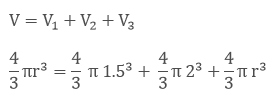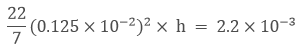• +91 9971497814
• info@interviewmaterial.com

# RD Chapter 16- Surface Areas and Volumes Ex-16.1 Interview Questions Answers

### Related Subjects

Question 1 : How many balls, each of radius 1 cm, can be made from a solid sphere of lead of radius 8 cm?

Given,
A solid sphere of radius, R = 8 cm
With this sphere, we have to make spherical balls of radius r = 1 cm
Let’s assume that the number of balls made as n
Then, we know that
Volume of the sphere = 4/3 πr3
The volume of the solid sphere = sum of the volumes of n spherical balls.
n x 4/3 πr3 = 4/3 πR3
n x 4/3 π(1)3 = 4/3 π(8)3
n = 83 = 512
Therefore, 512 balls can be made of radius 1 cm each with a solid sphere of radius 8 cm.

Question 2 : How many spherical bullets each of 5 cm in diameter can be cast from a rectangular block of metal 11dm x 1 m x 5 dm?

Given,

A metallic block ofdimension 11dm x 1m x 5dm

The diameter of eachbullet = 5 cm

We know that,

Volume of the sphere =4/3 πr3

Since, 1 dm = 10-1m= 0.1 m

The volume of therectangular block = 1.1 x 1 x 0.5 = 0.55 m3

Radius of the bullet =5/2 = 2.5 cm

Let the number ofbullets made from the rectangular block be n.

Then from thequestion,

The volume of therectangular block = sum of the volumes of the n spherical bullets

0.55 = n x 4/3 π(2.5)3

Solving for n, we have

n = 8400

Therefore, 8400 can becast from the rectangular block of metal.

Question 3 : A spherical ball of radius 3 cm is melted and recast into three spherical balls. The radii of the two of the balls are 2 cm and 1.5 cm respectively. Determine the diameter of the third ball?

Given,

Radius of thespherical ball = 3 cm

We know that,

The volume of thesphere = 4/3 πr3

So, it’s volume (V) =4/3 πr3

That the ball is meltedand recast into 3 spherical balls.

Volume (V1)of first ball = 4/3 π 1.53

Volume (V2)of second ball = 4/3 π23

Let the radius of thethird ball = r cm

Volume of third ball(V3) = 4/3 πr3

Volume of thespherical ball is equal to the volume of the 3 small spherical balls.Now,

Cancelling out thecommon part from both sides of the equation we get,

(3)3 =(2)3 + (1.5)+ r3

r= 33–23– 1.53 cm3

r=15.6 cm3

r = (15.6)1/3 cm

r = 2.5 cm

As diameter = 2 xradius = 2 x 2.5 cm

= 5.0 cm.

Thus, the diameter ofthe third ball is 5 cm

Question 4 : 2.2 cubic dm of brass is to be drawn into a cylindrical wire of 0.25 cm in diameter. Find the length of the wire?

Given,

2.2 dm3 ofbrass is to be drawn into a cylindrical wire of Diameter = 0.25 cm

So, radius of the wire(r) = d/2

= 0.25/2 = 0.125*10-2 cm

Now, 1 cm = 0.01 m

So, 0.1cm = 0.001 m

Let the length of thewire be (h)

We know that,

Volume of the cylinder= πr2h

It’s understood that,

Volume of cylindricalwire = Volume of brass of 2.2 dm3h = 448 m

Therefore, the lengthof the cylindrical wire drawn is 448 m

Question 5 : What length of a solid cylinder 2 cm in diameter must be taken to recast into a hollow cylinder of length 16 cm, external diameter 20 cm and thickness 2.5 mm?

Given,

Diameter of the solidcylinder = 2 cm

Length of hollowcylinder = 16 cm

The solid cylinder isrecast into a hollow cylinder of length 16 cm, external diameter of 20 cm andthickness of 2.5 mm = 0.25 cm

We know that,

Volume of a cylinder =πr2h

Radius of the solidcylinder = 1 cm

So,

Volume of the solidcylinder = π12h = πh cm3

Let’s assume thelength of the solid cylinder as h

And,

Volume of the hollowcylinder = πh(R2– r2)

Thickness of thecylinder = (R – r)

0.25 = 10 – r

So, the internalradius of the cylinder is 9.75 cm

Volume of the hollowcylinder = π × 16 (100 – 95.0625)

Hence, it’s understoodthat

Volume of the solidcylinder = volume of the hollow cylinder

πh = π × 16(100 –95.06)

h = 79.04 cm

Therefore, the lengthof the solid cylinder is 79.04 cm.

Question 6 : A cylindrical vessel having diameter equal to its height is full of water which is poured into two identical cylindrical vessels with diameter 42 cm and height 21 cm which are filled completely. Find the diameter of the cylindrical vessel?

Given,

The diameter of thecylinder = the height of the cylinder

h = 2r, where h –height of the cylinder and r – radius of the cylinder

We know that,

Volume of a cylinder =πr2h

So, volume of thecylindrical vessel = πr22r = 2πr3   (as h= 2r)….. (i)

Now,

Volume of eachidentical vessel = πr2h

Diameter = 42 cm, sothe radius = 21 cm

Height = 21 cm

So, the volume of twoidentical vessels = 2 x π 212 × 21 ….. (ii)

Since the volumes onequation (i) and (ii) are equal

On equating both theequations, we have

2πr3= 2 x π212 × 21

r3 =(21)3

r = 21 cm

So, d = 42 cm

Therefore, thediameter of the cylindrical vessel is 42 cm.

Question 7 : 50 circular plates each of diameter 14 cm and thickness 0.5 cm are placed one above the other to form a right circular cylinder. Find its total surface area.

Given,

50 circular plateseach with diameter 14 cm

Thickness of plates =0.5 cm

As these plates areone above the other, the total thickness of all the plates = 0.5 x 50 = 25 cm

So, the total surfacearea of the right circular cylinder formed = 2πr × h + 2πr2

= 2πr (h + r)

= 2(22/7) x 7 x (25 +7)

= 2 x 22 x 32 = 1408cm2

Therefore, the totalsurface area of the cylinder is 1408 cm2

Question 8 : 25 circular plates, each of radius 10.5 cm and thickness 1.6 cm, are placed one above the other to form a solid circular cylinder. Find the curved surface area and the volume of the cylinder so formed.

Given,

250 circular plateseach with radius 10.5 cm and thickness of 1.6 cm.

As the plates areplaced one above the other, the total height becomes = 1.6 x 25 = 40 cm

We know that,

Curved surface area ofa cylinder = 2πrh

= 2π × 10.5 × 40 =2640 cm2

And, volume of thecylinder = πr2h

= π × 10.5×40 = 13860 cm3

Therefore,

The curved surface areaof the cylinder is 2640 cm2 and the volume of the cylinder is13860 cm3

Question 9 : Find the number of metallic circular discs with 1.5 cm base diameter and of height 0.2 cm to be melted to form a right circular cylinder of height 10 cm and diameter 4.5 cm.

Given,

Radius of eachcircular disc = r = 1.5/2 = 0.75 cm

Height of eachcircular disc = h = 0.2 cm

Radius of cylinder = R= 4.5/ 2 = 2.25 cm

Height of cylinder = H= 10 cm

So, the number ofmetallic discs required is given by n

n = Volume of cylinder/ volume of each circular disc

n = πR2H/πr2h

n = (2.25)2(10)/(0.75)2(0.2)

n = 3 x 3 x 50 = 450

Therefore, 450metallic discs are required.

Question 10 : How many spherical lead shots each of diameter 4.2 cm can be obtained from a solid rectangular lead piece with dimensions 66 cm × 42 cm × 21 cm.

Given,

Radius of eachspherical lead shot = r = 4.2/ 2 = 2.1 cm

The dimensions of therectangular lead piece = 66 cm x 42 cm x 21 cm

So, the volume of aspherical lead shot = 4/3 πr3

= 4/3 x 22/7 x 2.13

And, the volume of therectangular lead piece = 66 x 42 x 21

Thus,

= 66 x 42 x 21/ (4/3 x22/7 x 2.13)

1500

Todays Deals### RD Chapter 16- Surface Areas and Volumes Ex-16.1 Contributorskrishan

Name:
Email:

# Latest News# 9000 interview questions in different categories